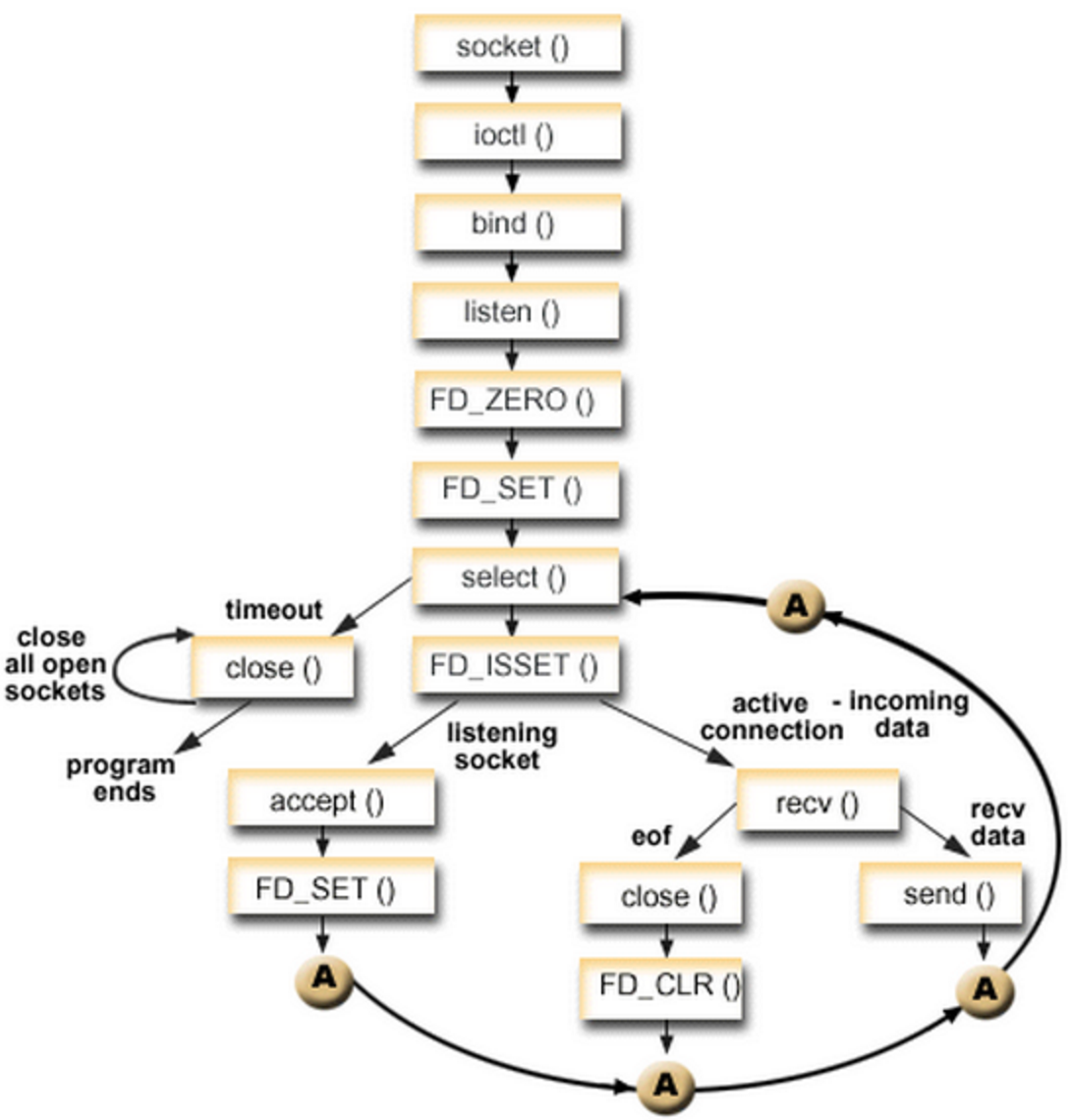# 多路复用IO----select

###//这是一个通过多路复用IO--select来实现的tcp服务端程序

#include<stdio.h>
#include<unistd.h>
#include<stdlib.h>
#include<string.h>
#include<errno.h>
#include<netinet/in.h>
#include<arpa/inet.h>
#include<sys/socket.h>

int main(int argc,char *argv[])
{
if(argc!=3)
{
printf("port ip\n");
return -1;
}
int sockfd=-1;
char buff={0};
int i=0;
int j=0;
int fd_arr={-1};//用于暂时存储文件描述符
int max_fd=0;
fd_set rds;
sockfd=socket(AF_INET,SOCK_STREAM,0);
if(sockfd<0)
{
perror("socket error\n");
return -1;
}
if(ret<0)
{
perror("bind error\n");
return -1;
}
if(listen(sockfd,5)<0)
{
perror("listen error\n");
return -1;
}//之前和正常服务端一样
for(i=0;i<1024;i++)
{
fd_arr[i]=-1;
}
fd_arr=sockfd;//把监听描述符特殊处理先放进队列
while(1)
{
max_fd=sockfd;
FD_ZERO(&rds);//初始化结构体
for( i=0;i<1024;i++)
{
if(fd_arr[i]!=-1)//如果数组中对应位置不为-1说明有文件描述符
{
FD_SET(fd_arr[i],&rds);//把文件描述符放入结构体
max_fd=max_fd>fd_arr[i]?max_fd:fd_arr[i];//更新最大描述符的值
}
}
struct timeval tv;
tv.tv_sec=3;
tv.tv_usec=0;
ret=select(max_fd+1,&rds,NULL,NULL,&tv);//这里我的文件描述符是读事件的集合所以后边两个位NULL
if(ret<0)//当返回值小于0代表失败
{
perror("select error\n");
continue;
}
else if(ret==0)//当返回值等于0代表超时了没有数据来
{
printf("no data arrived\n");
continue;
}
//返回值大于0代表正常这时候留在集合里边的都是已经就绪可以处理的文件描述符，返回的值就是文件描述符的个数
for( i=0;i<max_fd+1;i++)
{//没办法确定是哪个就绪了，所以需要遍历
if(FD_ISSET(i,&rds))//检查对应描述符是够可以读写如果可以继续判断
{
if(i==sockfd)//判断是不是监听描述符，因为因为监听描述符和别的描述符操作不一样
{//如果是监听描述符代表有新的链接来了。
int new_fd;
if(new_fd<0)
{
perror("accept error");
continue;
}
printf("have new connect \n");
for(j=0;j<1024;j++)
{
if(fd_arr[j]==-1)
{//把新连接的文件描述符放到暂存数据里边
fd_arr[j]=new_fd;
max_fd=max_fd>new_fd?max_fd:new_fd;
break;
}
}
}
else
{//如果不是监听描述符就接收数据
memset(buff,0x00,1024);
ret=recv(i,buff,1023,0);
if(ret<=0)
{
if(errno==EAGAIN||errno==EINTR)
continue;
}
perror("recv error");
close(i);//不是以上两个错误代表读完了就关闭掉对应描述符
for(j=0;j<1024;j++)
{//关掉描述符之后并把对应的数组中的描述符删掉
if(i==fd_arr[j])
{
fd_arr[j]=-1;
}
}
}
printf("client say:%s\n",buff);

}
}
}
close(sockfd);
return 0;
}
}


### 每次调用select的时候都需要把fd集合从用户态拷贝到内核态，这样会消耗大量资源。

03-142401-3020
10-16275
12-07761
06-284061
09-23972
07-2642
06-16503
11-0643